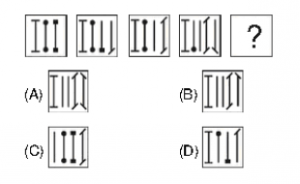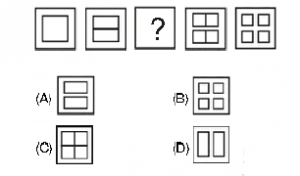# Picture Pattern Series

#### Video Lesson on Picture Pattern Series

Picture Pattern questions of Series are common part of Non-Verbal Reasoning in many of Aptitude tests like Armed Forces Commission Tests, NUST NET, and many other Pakistani Tests. The tests are specifically based on your ability to solve problems using pictures, rather than words. They are used by employers to determine which candidates have high levels of critical thinking, logical ability, mathematical ability and powers of deduction.

### Non verbal reasoning: Series Based Questions

This is the most common Non Verbal reasoning problem which is based on the continuation of figures. You will be provided with a series of images following a certain sequence. you have to choose one of the images from the set of options which follows the same sequence.

### Finding the next figure based questions.

In these questions, approx 4-5 problem figures are generally given. You have to observe the pattern followed by these problem figures and find out one of the answer figures from the options which continues the same pattern.

### Example

The figures given below follow a series. Out of these figures A, B, C and D which figure will replace the question mark so that the series remains continued?### Solution:

In each next figure the already presents lines start to deplete with the figures at their end being deleted. Also, a new line is added titled lined at its ends. The titled lines increase in each figure. Hence, the correct answer should be option (A).

### Finding the missing figure based questions.

These questions are similar to the above question, but in them, one figure from between the five problem figures is not given. You have to observe the pattern followed by these problem figures and find out one of the answer figures from the options which follows the same pattern.

### Example

In the following question what will come in place of the question mark so that the figures are in series.### Solution

The figures become half and become detached in the alternative figures. Hence the question make will be replaced by two rectangles aligned horizontally and at a distance from each other.

### About us

EntryTest.com is a free service for students seeking successful career.CAT - College of Admission Tests. All rights reserved. College of Admission Tests Online Test Preparation The CAT Online